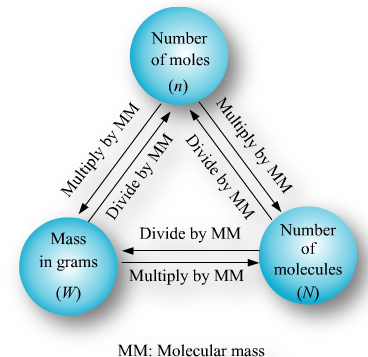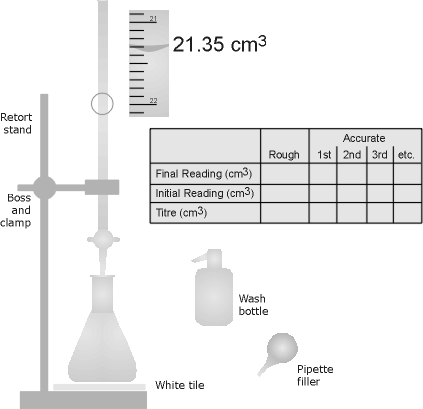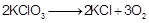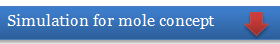×#### Thank you for registering.

One of our academic counsellors will contact you within 1 working day.

Click to Chat

1800-1023-196

+91-120-4616500

CART 0

• 0

MY CART (5)

Use Coupon: CART20 and get 20% off on all online Study Material

ITEM
DETAILS
MRP
DISCOUNT
FINAL PRICE
Total Price: Rs.

There are no items in this cart.
Continue Shopping

Mole Concept Of Physical chemistry class 11th,.................... .......................
Mole Concept Of Physical chemistry class 11th,....................      .......................


2 years ago

							 What is Mole?Atoms and molecules are too small to count. To solve this problem their numbers are expressed in terms of Avogadro’s number (NA = 6.023 1023).  Mole is the number equal to Avogadro’s number just like a dozen is equal to 12, a century means 100, a score means = 20.Mole can be defined as  a unit which represents 6.023 x1023 particles of same matter.A mole (symbol mol) is defined as the amount of substance that contains as many atoms, molecules, ions, electrons or any other elementary entities as there are carbon atoms in exactly 12 gm of. The number of atoms in 12 gm ofis called Avogadro’s number.One atomic mass unit (amu)The number of moles of a substance can be calculated by various means depending on data available, as follows.		Number of moles of moleculesNumber of moles of atomsNumber of moles of gases(Standard molar volume at STP = 22.4 lit)			Number of moles of particles e.g. atoms, molecules ions etcfor a compound, y moles of A = x moles of BMole fraction = fraction of the substance in the mixture expressed in terms of mol is called its mol fraction (X)	            E.g. for a mixture of substance A & B(n terms of denote number of moles)Refer to the following video for mole concept									Solved Examples													Question 1: Calculate the mass of (i) an atom of silver (ii) a molecule of carbon dioxide.			Solution:											1 mole of Ag atoms = 108 g= 6.022 x 1023 atoms			           6.022 x 1023 atoms of silver have mass = 108g			            Mass of one atom of silver1 mole of CO2 = 44 g= 6.022 x 1023 molecules			           Thus, 6.022 x 1023 molecules of CO2 has mass = 44 g			            1 molecule of CO2 has mass= 7.307 ´ 10-23 g			______________________________________________________________			Question 2:  Calculate the number of molecules present											in 34.20 grams of cane sugar (C12H22O11)																		in one litre of water assuming that the density of water is 1 g/cm3																		in one drop of water having mass 0.05 g.										Solution:											1 mole of C12H22O11 = 342 g= 12 x 12 + 22 x 1 + 11 x 16 = 342 amu			           = 6.022 x 1023 molecules			           Now 342 g of cane sugar contain 6.022 x 1023 molecules.			            34.2 g of cane sugar will contain= 6.022 x 1022 molecules											1 mole of water = 18 g = 6.022 x 1023 molecules.										           Mass of 1 litre of water = Volume x density = 1000 x 1 = 1000 g			           Now 18 g of water contains = 6.022 x 1023 molecules.			           1000 g of water will contain == 3.346 x 1025 molecules											1 mole of H2O = 18 g = 6.022 x 1023 molecules.										           Mass of 1 drop of water = 0.05 g			           Now 18 g of H2O contain = 6.022 x 1023 molecules.			           0.05 g of H2O will contain =________________________________________________			Question 3: Calculate the number of moles in each of the following:											392 grams of sulphuric acid																		44.8 litres of carbon dioxide at STP																		6.022 x 1023 molecules of oxygen (iv) 9.0 grams of aluminium (v) 1 metric ton of iron (1 metric ton = 103 kg ) (vi) 7.9 mg of Ca (vii) 65.5 mg of carbon.										Solution:											1 mole of H2SO4 = 98 gThus 98 g of H2SO4 = 1 mole of H2SO4			           392 g of H2SO4 == 4 moles of H2SO4											1 mole of CO2 = 22.4 litres at STP										           i.e. 22.4 litres of CO2 at STP = 1 mole			           44.8 litres of CO2 at STP == 2 moles CO2											1 mole of O2 molecules = 6.022 x 1023 molecules.										            6.022 x 1023 molecules = 1 mole of oxygen molecules.											1 mole of Al = 27 g of Ali.e. 27 g of aluminium = 1 mole of Al			            9 g of aluminium == 0.33 mole of Al											1 metric ton of Fe = 103 kg = 106 g										            1 mole of Fe = 56 g of Fe			            106 g of Fe == 1.786104 moles											7.9 mg of Ca = 7.910-3 g of CaEquivalent WeightEquivalent weight of a substance (element or compound) is defined as “The number of parts by weight of it, that will combine with or displace directly or indirectly 1.008 parts by weight of hydrogen, 8 parts by weight of oxygen, 35.5 parts by weight chlorine or the equivalent parts by weight of another element”.Equivalent weight of any substance depends on the reaction in which it takes part.Equivalent weight is a relative quantity so it is unit less. When equivalent weight of a substance is expressed in grams, it is called Gram Equivalent Weight (GWE).Calculation of Equivalent WeightEquivalent weight  = Molar mass  / Valence factor		Valence factor for base = acidity of base			Valence factor for acid = basicity of acid			Valence factor for element = valencyTitrationTitration is a procedure of determining the concentration of unknown solution with the help of solution of known concentration.In this procedure of determining the concentration of solution A by adding carefully measured volumes of a solution of known concentration B until the reaction of A with B is just complete.Law of equivalence:The fundamental basis of titration is the Law of Equivalence which states that“at end point of a titration, volumes of the two titrants reacted have the same number of equivalents or mili equivalents”Acid Base Titration:One gram equivalent of acid neutralized by one gm equivalent of base. It meansOne equivalent of Acid = One Equivalent of BaseAcid [N1V1] = Base [N2V2][Gram equivalent = Normality x Volume]									Example													Quesion			Find the number of milli equivalents of H2SO4 present in 10 mL of N/2 H2SO4 solution.			Solution			Milli equivalents = Normality x Volume (mL) = ½ X 10 = 5 milli equivalent of H2SO4						Limiting ReactantThe reactant which is totally consumed during the course of reaction and when it is consumed reaction stops.The concept of limiting reactant is applicable to reaction other than monomolecular i.e., when more than one type reactant involved. For example. These is no limiting reactant.To determine the limiting reagent amount of all reactants and mole ratio of reactants must be known. If the ratio of moles of reactant A with respect to reactant B is greater than the ratio of the moles of A to moles of B for a balanced chemical equation then B is the limiting reactant.All other terms like left (unused) mass of other reactant, amount of formed product can be known stoichiometrically by knowing the amount of limiting reactant.Question 1:  The largest number of molecules is in:a. 28 g of COb. 46 g of C2H5OHc. 36 g of H2Od. 54 g of N­2O5                         Question 2: The number of molecules in 89.6 litre of a gas at NTP are:a. 6.02 × 1023b. 2 × 6.02 × 1023c. 3 × 6.02 × 1023d. 4 × 6.02 × 1023Question 3: The total number of protons in 10 g of calcium carbonate is:a. 3.0115 × 1024b. 1.5057 × 1024c. 2.0478 × 1024d. 4.0956 × 1024Question 4: What is equivalent weight of NaCl?a. 58.5b. 12.12c. 13.45d. 65.42Q.1									Q.2									Q.3									Q.4													c									d									a									a

2 years ago
Think You Can Provide A Better Answer ?

## Other Related Questions on Physical Chemistry

View all Questions »### Course Features

• 731 Video Lectures
• Revision Notes
• Previous Year Papers
• Mind Map
• Study Planner
• NCERT Solutions
• Discussion Forum
• Test paper with Video Solution### Course Features

• 141 Video Lectures
• Revision Notes
• Test paper with Video Solution
• Mind Map
• Study Planner
• NCERT Solutions
• Discussion Forum
• Previous Year Exam Questions